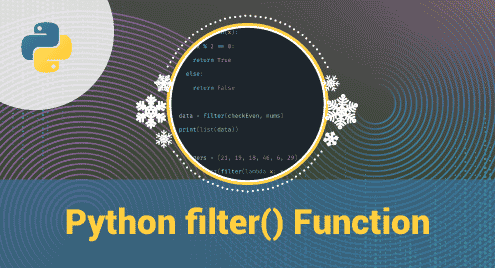Scripting

# Python filter() Function## Introduction

The filter() function helps the user to filter a sequence of elements according to the given condition to check if it is true or not. This function helps to filter elements in the sequence faster and more accurately. And the filtered-out elements are sorted in the original order.

Now we’re gonna guide you about using the filter() function in Python. Hope you understand.

## Example

Filter numbers less than or equal to 6:

```num = [3, 7, 5, 9, 1, 2, 8, 6]

def func(x):

if x > 6:

return False

else:

return True

number = filter(func, num)

for x in number:

print(x)```

Output:
3

5

1

2

6

## Definition

The filter() function prints the result according to the accepted condition.

## The syntax

`filter(function, iterable)`

Parameter Values:

function: function to check element

iterable: sequence to be filtered

## More examples

Example 1: Filter even numbers

```num = [3, 7, 5, 9, 1, 2, 8, 6]

def even(x):

if x % 2 == 0:

return True

else:

return False

number = filter(even, num)

for x in number:

print(x)```

Output:
2

8

6

Example 2: filters vowels

# filters vowels

```def vowel(x):

vowels = ['a', 'e', 'i', 'o', 'u']

if (x in vowels):

return True

else:

return False

# sequence

letters = ['a', 'g', 'h', 'i', 'k']

# using filter()

filtered = filter(vowel, letters)

for x in filtered:

print(x)```

Output:
a

i

## Conclusion

And we guided you on how to use the filter() function in Python.

Thank you for checking it out!